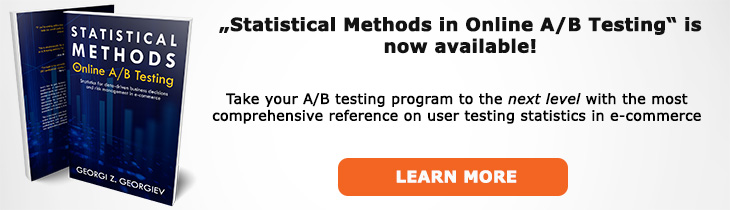# What does "Alternative Hypothesis" mean?

Definition of Alternative Hypothesis in the context of A/B testing (online controlled experiments).

## What is an Alternative Hypothesis?

The alternative hypothesis is usually a claim we hope to be true, e.g. the tested variant is performing better than the control group, the treatment is outperforming the current experience by X%, etc. Once we have an alternative we can select the null hypothesis such that the null and alternative exhaust the entire parameter space (Θ0 ∪ Θ1 = Θ, Θ0 ∩ Θ1 = ∅).

The alternative hypothesis is usually denoted by H1 (H2, etc. in case there are multiple alternatives under consideration).

Most often in A/B tests we set up a superiority alternative such as H1: μ > 0 or H0: μ > ε where ε is some positive discrepancy. However, sometimes the alternative is that of non-inferiority so it is H0 > -ε where ε is the magnitude of the non-inferiority margin.

Upon observing a p-value below the significance threshold we selected we can then reject the null hypothesis and accept the alternative. One should remember that accepting the alternative means exactly that and not that any particular sub-set or point value from the alternative space is accepted. For example observing a percentage difference in conversion rate of 5% in the treatment group versus the control group and having a p-value less than the significance threshold does not mean that we accept the alternative: "the conversion rate of the treatment group is 5% higher" or "the conversion rate of the treatment group is 5% higher or more" if the p-value was calculated under a null of "the percentage difference between the treatment group and control group is less than or equal to zero". Only if the p-value was calculated under a null hypothesis which compliments these specific alternative hypotheses can one make these claims.

## Related A/B Testing terms

Type II Error

Hypothesis

One-Sided Hypothesis

Two-Sided Hypothesis

Like this glossary entry? For an in-depth and comprehensive reading on A/B testing stats, check out the book "Statistical Methods in Online A/B Testing" by the author of this glossary, Georgi Georgiev.## Glossary Index by Letter

Select a letter to see all A/B testing terms starting with that letter or visit the Glossary homepage to see all.